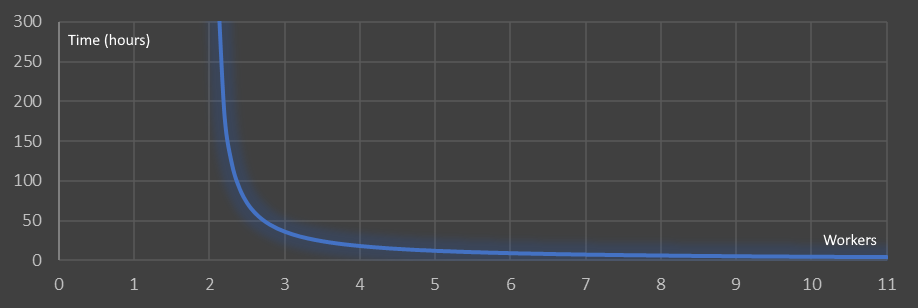# Toxic Sludge Puzzle

Let’s imagine you work in a factory that handles toxic sludge.
One day, an accident occurs and a pipe breaks. This causes a continuous leak of sludge. The sludge leaks with a constant (unknown) rate. Luckily for you there is a pit underneath which contains the sludge. The break occurred an unknown time ago so you also do not know the current volume of liquid in the pit.Unlucky for you, the cut-off switch to stop the flow of sludge is at the bottom of the pit. To get to the switch you need to remove all the sludge from the pit.
You can use an army of people (all suitably attired in appropriate protective equipment) to empty the pit. Each person can safely remove one gallon of sludge per hour.If five people can empty the pit in twelve hours, and eleven people can empty the pit in four hours, how many people would it take to empty the pit in an hour?
How many people are needed to empty the pool in an hour?

## Rate Puzzle

If the leak were not present, this would be an easier problem, but because the leak is continuous, the longer you take, the more sludge you have to remove.
There are two unknowns in this puzzle (the volume of fluid in the pit, and the rate of inflow from the damaged pipe). Thankfully we also have two equations involving these values, so we have sufficiently information to solve for the unknowns.
If V is the volume of sludge in the pit at the start, and r is the rate of inflow from the pipe, then we can write two equations from the data given: The initial volume of sludge, less the number of people multiplied by the hours worked, plus the number of hours multiplied by the inflow. This must be equal to zero to get access to the switch (the pit is empty).V − (5 × 12) + 12r = 0
V − (4 × 11) + 4r = 0
The two simultaneous equations are:
V + 12r = 60
V + 4r = 44
Subtracting these two gives the result that r = 2. The rate of inflow of sludge is two gallons per hour.
Substituting this back in we can see that V = 36. There are 36 gallons of sludge in the pit at the start of the clean-up.
If we wanted to clean-up the mess in one hour, the we’d need to clean up 36+2 gallons (the initial sludge, plus the two gallons that flow in during the hour of clean up). This means we’d need 38 people.
38 people could clean-up the leak in an hour.

## Graph

Here is a graph of the function (number of people on the x-axis, and time on the y-axis). People are quantized, you can’t have a fraction of a person (though I guess you could ask people to not work a full hour).It’s obvious from the graph (and the formula, and common sense), that if you have less than two workers you are never going to empty the pit (the inflow is faster than the outflow). With two workers, you are treading water. If you throw more workers at the problem there is a double bonus; not only do you get the job finished quicker but, because you take less time, less sludge flows in, so there is less to move.